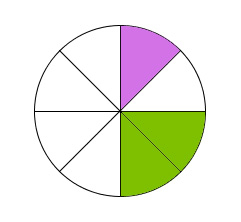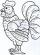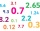# What is 11

What is the quotient of Three-fifths and 1 Over 10?

K =  6

### Step-by-step explanation:Did you find an error or inaccuracy? Feel free to write us. Thank you!Tips to related online calculators
Need help to calculate sum, simplify or multiply fractions? Try our fraction calculator.
Do you want to perform natural numbers division - find the quotient and remainder?

## Related math problems and questions:

• A quotientWhat is the quotient of 3/10 divided by 2/4 as a fraction?
• Quotient and divisionWhat is the quotient of 2/9 and 1/8?
• FractionsSort fractions z1 = (6)/(11); z2 = (10)/(21); z3 = (19)/(22) by its size. Result write as three serial numbers 1,2,3.
• QuotientDetermine the quotient (q) and the remainder (r) from division numbers 100 and 8. Take the test of correctness.
• Quotient and divisionFind the quotient of 3/4 and 1/4.
• Quotient 3If the quotient of 8/13 and 2 is subtracted from the product of 1 3/4 and 8/21, what is the difference?
• Two numbers and its productThe product of two numbers are 2/3. If on of them is 1/10, what is the other?
• Evaluate 11Multiply the quotient of 6 and 2 by 3, then add 1. Add 1 and 6, then divide by 2 and multiply by 3. Divide 6 by the product of 2 and 3, then add 1.Why does 1 3/4 + 2 9/10 equal 4.65? How do you solve this?
• Sum three fractionsWork out the sum of 1/4, 1/5 and 3/10.
• What is 9What is the value of x in the proportion 2 and one-fourth over x = 1 and one-half over 3 and three-fifths? 2 and two-fifths 5 and two-fifths 8 and 1 over 10 12 and 3 over 20Add two mixed fractions: 2 4/6 + 1 3/6If the quotient of [8/5 divided by 8/10] is added to the product of [8/14 x 7/12 x 3/8], what is the sum?A shopkeeper sells 8 1/3 kg, 10 1/4 kg and 11 1/5 kg of apples on 3 consecutive days. What is the total weight of apples sold?What is 1/3 as a decimal? Give your answer rounded to 2 decimal places.The length of 12 pipes is 10 1/2 meters. (1) find the length of one pipe (2) also find the length of 7 pipes1/3 of a cake shared with 4 people. What share of the whole cake has each people?